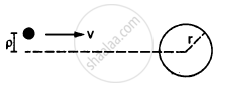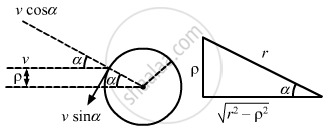Advertisement Remove all ads

# A Small Particle Travelling with a Velocity V Collides Elastically with a Spherical Body of Equal Mass and of Radius R Initially Kept at Rest. - Physics

Sum

A small particle travelling with a velocity v collides elastically with a spherical body of equal mass and of radius r initially kept at rest. The centre of this spherical body is located a distance ρ(< r) away from the direction of motion of the particle (see figure below). Find the final velocities of the two particles.[Hint : The force acts along the normal to the sphere
through the contact. Treat the collision as onedimensional
for this direction. In the tangential direction no force acts and the velocities do not change].

Advertisement Remove all ads

#### Solution

It is given that the mass of both the bodies (small particle and spherical body) is same.
Let the velocity of the particle be v.

We break the particle velocity into two components: v cosα (normal to the sphere) and v sinα (tangential to the sphere).From figure,
cosα $= \sqrt{r^2 - \rho^2}$ and sinα $= \frac{\rho}{r}$
Here, the collision occurs due to the component v cosα.

After an elastic collision, bodies of same mass exchange their respective velocities.
Thus, the spherical body will have a velocity v cosα, while the particle will not have any component of velocity in this direction.

However, the tangential velocity of the particle v sinα will remain unaffected.

Thus, we have:
Velocity of the sphere = v cosα
$= \frac{v}{r}\sqrt{r^2 - \rho^2}$
And,
Velocity of the particle = v sinα $= \frac{v\rho}{r}$

Concept: Centre of Mass of a Rigid Body
Is there an error in this question or solution?
Advertisement Remove all ads

#### APPEARS IN

HC Verma Class 11, 12 Concepts of Physics 1
Chapter 9 Centre of Mass, Linear Momentum, Collision
Q 64 | Page 164
Advertisement Remove all ads
Advertisement Remove all ads
Share
Notifications

View all notifications

Forgot password?